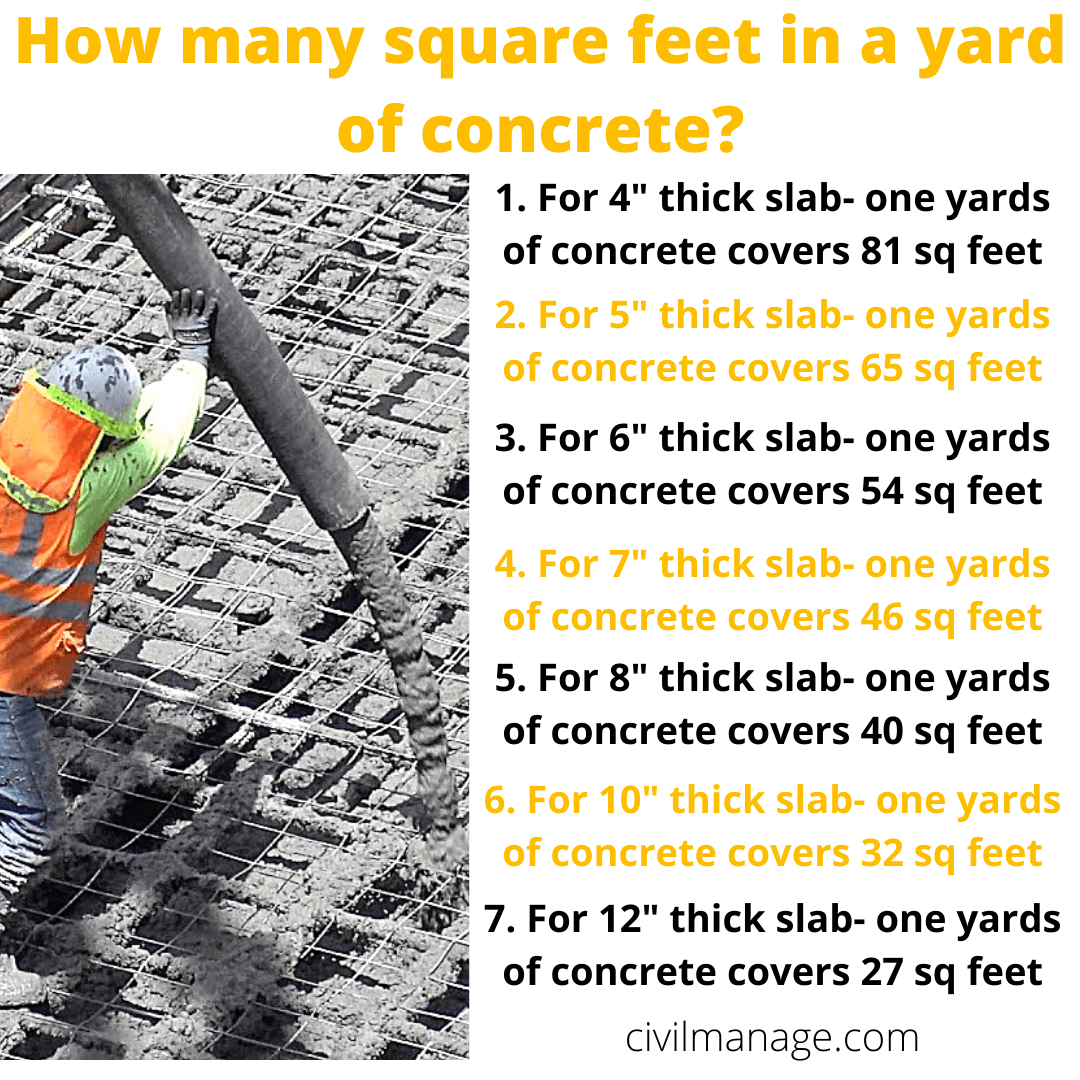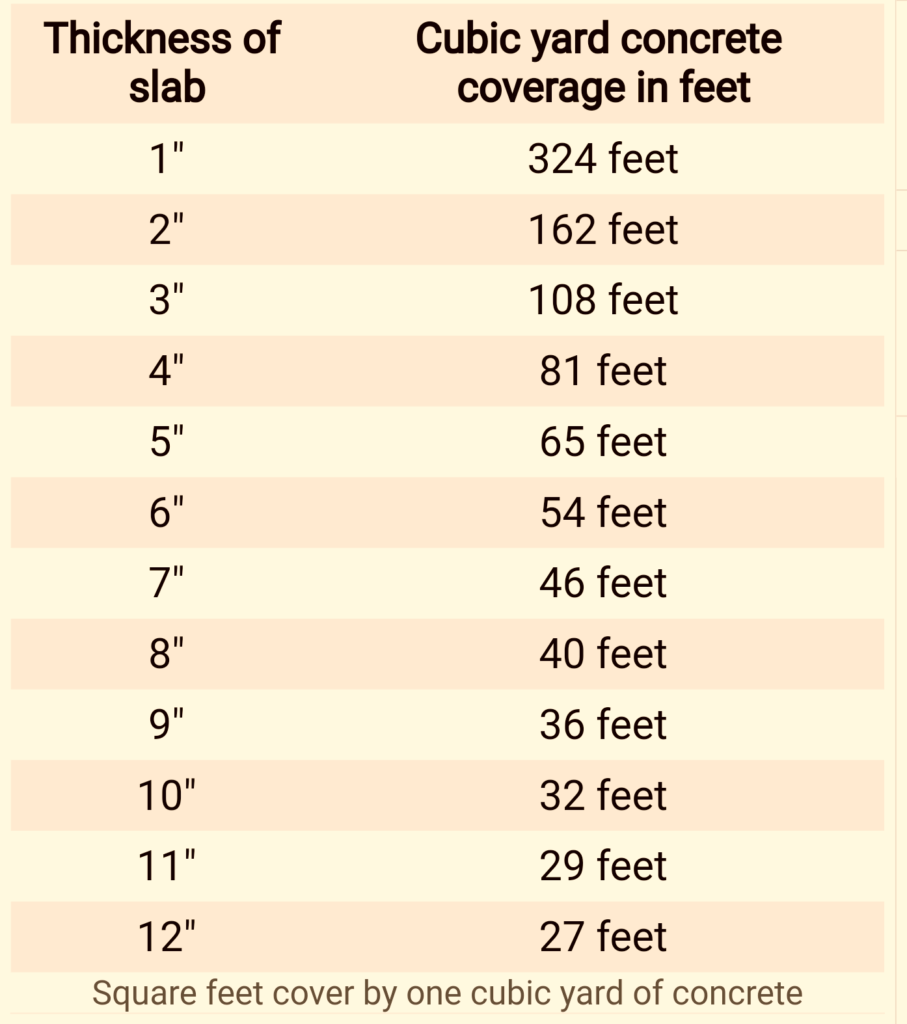# How many Square Feet in a yard of concrete?

Do you want to construct a patio or slab but don’t know how many bags of concrete do you need? Don’t worry this article is about how many square feet in a yard of concrete or how many sq feet does a yard of concrete covers?

Volume of ready mix concrete is measured in cubic yards. If you know the length and width of area and thickness of patio/slab as well. You can calculate that how much concrete you need for your project?

## How many square feet in a yard of concrete?

If you are looking for an answer about how many sq feet does a cubic yard of concrete covers? coverage of yardage of concrete depends on the thickness of slab. Simply for 1 inch thick slab, 1 cubic yard of concrete covers 324 square feet. Table below shows the coverage of one cubic yard of concrete for different thickness.

## How many square feet does a yard of concrete covers?

Following are the square feet area that one cubic yards of concrete covers at certain thickness of slab. If You are seeking for answer that “How much is a yard of concrete?”. Below we mentioned how much a single yard of concrete can help you for different thickness.

1. For 4 inches thick slab, 1 yard of concrete covers 81 square feet.
2. 5 inches thick slab, 1 yard of concrete covers 65 square feet.
3. For 6 inches thick slab, 1 yard of concrete covers 54 sq feet.
4. 7 inches thick slab, 1 yard of concrete covers 46 sq feet.
5. 8 inches thick slab, 1 yard covers 40 sq feet.
6. 10 inches thick slab, 1 yard covers 32 square feet.
7. 12 inches thick slab, 1 yard of concrete covers 27 sq feet.
See also  Types of concrete and types of concrete finish?

Read also concrete calculation

### How to calculate square feet cover by one cubic yard of concrete?

If you looking for how much cubic yards of concrete do you need? It is simple mathematics formula, measure your depth of slab/patio. Convert it into foot and divide 27 by that depth (foot), because there’s 27 cubic feet in 1 cubic yards.

Ok lets calculate the how many sq feet does a yard of concrete covers?

1. Suppose the depth of slab/patio is 9″. Convert it into foot 9″/12 = 0.75′.
2. Now divide 27 by 0.75 = 36 sq feet.
3. This calculation shows that 1 cubic yards of concrete will cover 36 square feet of 9″ slab.

Related: How much concrete do you need?

## How many yards of concrete do you need for 4 inch thick slab:

Slab and patios are almost 4 inches this. Below we will calculate how many cubic yards of concrete do you need for different slab areas with 4 inch thickness. As 1 cubic yard of concrete cover 81 square feet at 4 inch thickness.

1. 8′ × 10′ slab with 4 inch thickness 80/81 = 0.897 cubic yard of concrete.
2. 10′ × 10′ slab with 4 inch thickness 100/81 = 1.23 cubic yard of concrete.
3. 10′ × 12′ slab with 4 inch thickness 120/81 = 1.48 cubic yard of concrete.
4. 12′ × 12′ slab with 4 inch thickness 144/81 = 1.77 cubic yard of concrete.
5. 10′ × 14′ slab with 4 inch thickness 140/81 = 1.72 cubic yard of concrete.
6. 12′ × 14′ slab with 4 inch thickness 168/81 = 2.07 cubic yard of concrete.
7. 14′ × 14′ slab with 4 inch thickness 196/81 = 2.41 cubic yard of concrete.
8. 20′ × 20′ slab with 4 inch thickness 400/81 = 4.93 cubic yard of concrete.
9. 24′ × 24′ slab with 4 inch thickness 576/81 = 7.11 cubic yard of concrete.

## How many yards of concrete do you need for 6 inch thick slab:

Below is calculation how many cubic yards of concrete do you need for different slab areas with 6 inches thickness. As 1 cubic yard of concrete cover 54 square feet at 6 inch thickness.

1. 8′ × 10′ slab with 6 inch thickness 80/54 = 1.48 cubic yard of concrete.
2. 10′ × 10′ slab with 6 inch thickness 100/54 = 1.85 cubic yard of concrete.
3. 10′ × 12′ slab with 6 inch thickness 120/54 = 2.22 cubic yard of concrete.
4. 12′ × 12′ slab with 6 inch thickness 144/54 = 2.66 cubic yard of concrete.
5. 10′ × 14′ slab with 6 inch thickness 140/54 = 2.59 cubic yard of concrete.
6. 12′ × 14′ slab with 6 inch thickness 168/54 = 3.11 cubic yard of concrete.
7. 14′ × 14′ slab with 6 inch thickness 196/54 = 3.62 cubic yard of concrete.
8. 20′ × 20′ slab with 6 inch thickness 400/54 = 7.40 cubic yard of concrete.
9. 24′ × 24′ slab with 6 inch thickness 576/54 = 10.66 cubic yard of concrete.
See also  Building construction: Building construction typeshow many square feet in a yard of concrete

## How many yards of concrete do You need?

Below we will guide you, how do you calculate how many yards of concrete you need? From the above discussion and table you may get the depth of patio/slab and how much 1 yard concrete covers. How many square feet in a yard of concrete 5 inches thick? 5 inch thick slab cover 65 square feet by one cubic yard of concrete.

1. Thickness = 5″.
2. Measure the area by length×width = 12×12 = 144 sq ft.
3. Divide 144 ÷ 65 = 2.21 cubic yards of concrete you need.

As for 5″ thickness, 1 yard of concrete covers the 65 sq feet, so we divided the area (144) by 65. If there’s 6″ thickness we divide area by 54 and 46 for 7″ and so on.

### How much cement do You need for a 12×12 slab?

If you are seeking answer about ” How many concrete bags do You need for 12×12 slab?”. For 4″ formula is Length × width × thickness (in feet). Then divide this cubic feet volume by 35.64 to convert into cubic yards. For 60lb concrete bag further divide 0.0167, for 80lb bag divide by 0.222.

• 12 × 12 × 0.33 = 47.54 cft.
• 47.54 ÷ 35.64 = 1.33 cubic yards.
• For 60lb bag 1.33 ÷ 0.0167 = 80 bags.
• For 80lb bag 1.33 ÷ 0.222 = 61 bags.

### How many yards of concrete do You need for a 24x24x4 slab:

To calculate how much concrete do you need for 24’×24’×4″ slab, is a simple maths. As for 4″ thick one cubic yard of concrete covers 81 square feet, simply divide the area by 81. You will get how much concrete you really need?

For 4″ thickness the formula is (length × width) ÷ 81.

1. Lets calculate the area 24’×24’=576 square feet.
2. Divide 576 ÷ 81 = 7.11 cubic yards.
3. You need 7.11 cubic yard of concrete for 24×24×4 slab.

For 6″ thickness the formula is (length × width) ÷ 54.

1. Area 24′ × 24′ = 576 square feet.
2. Divide 576 ÷ 54 = 10.66 cubic yards.

### How many yards of concrete do I need for a 10×10 slab?

Lets calculate how much concrete do you need for 10’×10′ slab for 4″ thickness & 6″ thickness..

1. Formula for 4″ = Length × width ÷ 81.
2. Area 10′ × 10′ = 100 square feet.
3. Divide 100 ÷ 81 = 1.23 square yards of concrete you need for 10’×10’×4″ slab.

1. Formula for 6″ = Length × width ÷ 54.
2. Area 10′ × 10′ = 100 sq feet.
3. Divide 100 ÷ 54 = 1.85 square yards of concrete you need 10′ × 10′ × 4″ slab.

### How many yards of concrete for 1500 sq ft?

For different thickness you need different yards of concrete 1500 sq ft. Let say

1. If the thickness is 4″, simply 1500 ÷ 81 = 18.51 cubic yards.
2. For 6″ Thickness 1500 ÷ 54 = 27.77 yards.
3. Same as if 8″ thick 1500 ÷ 40 = 37.5 yards.

### How many square feet in a yard of concrete 8 inches thick?

One cubic yard of concrete covers 40 square feet if the slab/patio thickness is 8″.

## How many square feet in a yard of concrete 6 inches thick?

Lets calculate the one cubic yard of concrete coverage area in sq feet for 6 inches thick slab/patio. One cubic yard of concrete has 54 square feet coverage for 6 inch thickness. See below for the calculation.

1. Lets suppose thickness= 6″ = 0.5′.
2. Now divide 27 by 0.5 = 27/0.5 = 54 square feet.
3. One cubic yard of concrete will cover 54 sq feet for 6″ thickness.

### How many square feet will 10 yards of concrete cover?

The coverage of one yard of concrete is different for each thickness. For example 1 yard concrete covers 324 square feet, so the 10 yards will cover 3240 sq ft. For more thickness just multiply the 10 yards with per yard coverage.

1. For 4″ thickness 10 × 81= 810 sq ft.
2. If its 6″ thick 10 × 54 = 540 sq ft

## How many square feet in a yard of concrete 4 inches thick?

Lets calculate the one cubic yard of concrete coverage area in sq feet for 4 inches thick slab/patio. One cubic yard of concrete has 81 square feet coverage for 4 inch thickness. See below for the calculation.

1. Lets suppose thickness= 4″ = 0.33′.
2. Now divide 27 by 0.33 = 27/0.33 = 81 square feet.
3. One cubic yard of concrete will cover 81 sq feet for 4″ thickness.

## How do you calculate square feet into yards of concrete?

1. Length × with × thickness. (Thickness should be in feet, let suppose 4″)
2. 10 × 12 × 0.33 = 40 Cubic feet
3. 40 × 0.037 = 1.48 cubic yard of concrete you need for 10’×10’×4″.

NOTE: 1 cubic feet = 0.037 cubic yard, so we multiply the volume by 0.037 to get cubic yardage.

#### Conclusion: Do You still have query?

Above we explained everything related to How many square feet does a cubic yard of concrete cover at different thickness. If you still have any query & questions please feel free to ask in comments.

Share